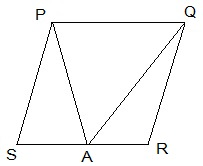# A farmer was having a field in the form of a parallelogram PQRS. She took any point A on RS and joined it to points P and Q. In how many parts the fields is divided? What are the shapes of these parts? The farmer wants to sow wheat and pulses in equal portions of the field separately. How should she do it?Solution

The field is divided into three parts each in triangular shape.

Let us assume,

ΔPSA, ΔPAQ and ΔQAR be the triangles.

Area of (ΔPSA + ΔPAQ + ΔQAR) = Area of PQRS — (i)

Area of ΔPAQ = ½ area of PQRS — (ii)

From the diagram we observe that the triangle and parallelogram are on the same base and in-between the same parallel lines.

From (i) and (ii) we get,

Area of ΔPSA + Area of ΔQAR = ½ area of PQRS — (iii)

From (ii) and (iii), we can conclude that,

The farmer must sow wheat or pulses in ΔPAQ or either in both ΔPSA and ΔQAR.(12)(5)